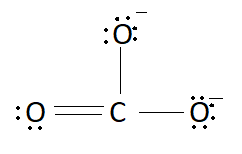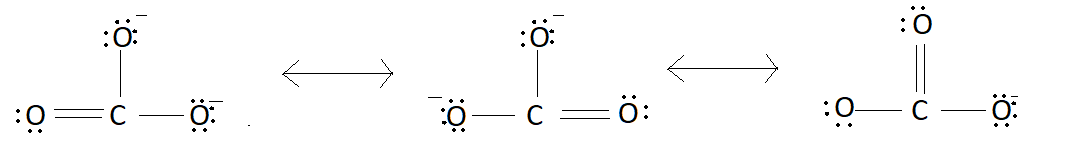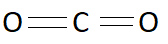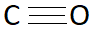QuestionAnswers

# The correct order of increasing $C - O$ bond length in $CO$, $C{O_2}$ and $CO_3^{2 - }$ is:A: $CO_3^{2 - } < C{O_2} < CO$B: $CO < C{O_2} < CO_3^{2 - }$C: $CO_3^{2 - } < CO < C{O_2}$D: $CO = C{O_2} = CO_3^{2 - }$

Hint: Bond length is defined as the average distance between nuclei of two bonded atoms in a molecule. Bond length depends upon the bond order. Generally it is approximately equal to the sum of covalent radii of two atoms.

We know bond length is the average distance between the nuclei of two atoms that are bonded together with a bond. Bond order affects the bond length between two atoms. More will be the value of bond order smaller will be the bond length. Bond order is calculated by taking the ratio of total number of bonds to the total number of canonical forms. Canonical forms are the resonating structures of a molecule which are formed by the delocalization of charge. Structure of $CO_3^{2 - }$ is:There will be resonance in this structure. Charge on oxygen atoms will keep on moving. As shown in the pictures:These are the resonating structures of $CO_3^{2 - }$. We know bond order is the ratio of number of bonds to resonating structures. In $CO_3^{2 - }$ total bonds are $4$ and resonating structures are $3$. Therefore bond order of $CO_3^{2 - }$ is:
Bond order$= \dfrac{4}{3} = 1.33$
Structure of $C{O_2}$ is:Since there is no charge there will be no other resonating structure of $C{O_2}$. This has only one structure. So bond order will be the ratio of total number of bonds to the total number of bonding molecules. Number of bonds in $C{O_2}$ are $4$ and the number of bonding molecules is $2$. So the bond order will be:
Bond order$= \dfrac{4}{2} = 2$
Structure of $CO$is:Like$C{O_2}$, $CO$ also does not possess any charge. This means $CO$ also has only one structure. Number of bonds in $CO$ is $3$ and the number of bonding molecules is $1$ . So the bond order will be:
Bond order$= \dfrac{3}{1} = 3$
As we know more the value of bond order less will be the bond length. Among given molecules bond order for $CO$is highest this means bond length of $CO$ will be least and for $CO_3^{2 - }$ value of bond order is least this means bond length of $CO_3^{2 - }$ will be the greatest. Therefore order for bond length will be $CO < C{O_2} < CO_3^{2 - }$
So the correct answer is option B that is $CO < C{O_2} < CO_3^{2 - }$.

Note:
Like bond length, bond angle is also different for different molecules. For the formation of bond angle there must be at least three atoms and two bonds. Bond angle is the angle which is formed between three atoms across at least two bonds.